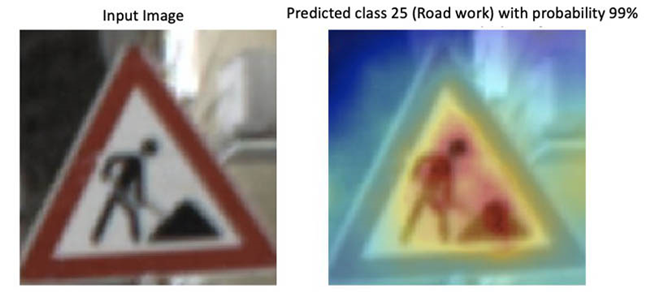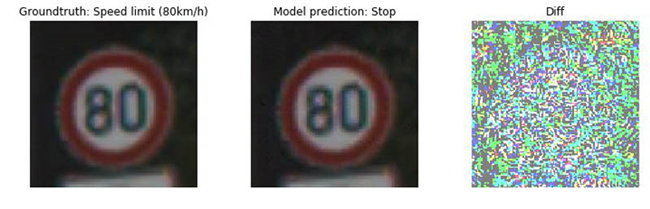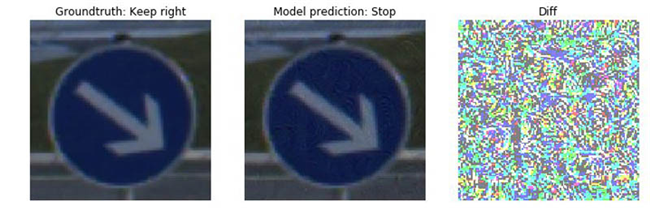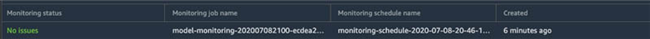# 使用 Amazon SageMaker Model Monitor 与 Debugger 检测并分析不正确的模型预测结果

Original URL: https://aws.amazon.com/cn/blogs/machine-learning/detecting-and-analyzing-incorrect-model-predictions-with-amazon-sagemaker-model-monitor-and-debugger/## 定义一个SageMaker模型

``sagemaker_session.upload_data(path='model.tar.gz', key_prefix='model')``

``````from sagemaker.pytorch.model import PyTorchModel

model = PyTorchModel(model_data = 's3://' + sagemaker_session.default_bucket() + '/model/model.tar.gz',
role = role,
framework_version = '1.5.0',
source_dir='entry_point',
entry_point = 'pretrained_model.py',
py_version='py3')``````

## 设置Model Monitor并部署模型

Model Monitor会自动监控生产环境中的机器学习模型，并在检测到数据质量问题时向您发出警报。在这套解决方案中，我们将捕捉端点的输入与输出，并创建监控计划以保证Model Monitor能够检查收集到的数据以及相关模型预测结果。我们使用`DataCaptureConfig` API指定Model Monitor在目标Amazon S3存储桶上存储模型输入与输出的比例。在以下示例中，采样百分比设置为50%：

``````from sagemaker.model_monitor import DataCaptureConfig

data_capture_config = DataCaptureConfig(
enable_capture=True,
sampling_percentage=50,
destination_s3_uri='s3://' + sagemaker_session.default_bucket() + '/endpoint/data_capture'
)``````

``````predictor = model.deploy(initial_instance_count=1,
instance_type='ml.m5.xlarge',
data_capture_config=data_capture_config)

endpoint_name = predictor.endpoint ``````

## 对测试图像进行推理

``````#invoke payload
response_body = response['Body']

#get results## 创建一项Model Monitor计划

``````monitor = ModelMonitor(
role=role,
image_uri='%s.dkr.ecr.us-west-2.amazonaws.com/sagemaker-processing-container:latest' %my_account_id,
instance_count=1,
instance_type='ml.m5.xlarge',
env={'THRESHOLD':'0.5'}
)``````

``````from sagemaker.model_monitor import CronExpressionGenerator
from sagemaker.processing import ProcessingInput, ProcessingOutput

destination = 's3://' + sagemaker_session.default_bucket() + '/endpoint/monitoring_schedule'
processing_output = ProcessingOutput(output_name='model_outputs', source='/opt/ml/processing/outputs', destination=destination)
output = MonitoringOutput(source=processing_output.source, destination=processing_output.destination)

monitor.create_monitoring_schedule(
output=output,
endpoint_input=predictor.endpoint,
schedule_cron_expression=CronExpressionGenerator.hourly()
)``````

``````for file in files:
for entry in content.split('\n'):

## 捕获意外模型行为``````Warning: Class 14 ('Stop sign') predicted more than 80 % of the time which is above the threshold
Predicted classes {9: 2, 19: 2, 25: 1, 14: 322, 13: 5, 5: 1, 8: 10, 18: 1, 31: 4, 26: 8, 33: 4, 36: 4, 29: 20, 12: 8, 22: 4, 6: 4}``````

## 创建Debugger hook配置

``'.*bn|.*bias|.*downsample|.*ResNet_input|.*image'``

``````def model_fn(model_dir):

model = resnet.resnet18()
model.eval()

#hook configuration
save_config = smd.SaveConfig(mode_save_configs={
smd.modes.PREDICT: smd.SaveConfigMode(save_interval=1)
})

hook = Hook("s3://" + sagemaker_session.default_bucket() + "tensors",
save_config=save_config,
include_regex='.*bn|.*bias|.*downsample|.*ResNet_input|.*image' )

#register hook
hook.register_module(model)

#set mode
hook.set_mode(modes.PREDICT)

return model ``````

``````model = PyTorchModel(model_data = 's3://' + sagemaker_session.default_bucket() + '/model/model.tar.gz',
role = role,
framework_version = '1.3.1',
source_dir='code',
entry_point = 'pretrained_model_with_debugger_hook.py',
py_version='py3')``````

``````predictor = model.deploy(
instance_type = 'ml.m5.xlarge',
initial_instance_count=1,
endpoint_name=endpoint_name,
data_capture_config=data_capture_config,
update_endpoint=True)``````

## 使用Debugger分析不正确预测

``````from smdebug.trials import create_trial

trial = create_trial('s3://' + sagemaker_session.default_bucket() + '/endpoint/tensors')``````

``trial.tensor('ResNet_bn1.bias').value(step_num=0, mode=modes.PREDICT).``

### 计算隐性偏差

``````weight = trial.tensor(weight_name).value(step_num=step, mode=modes.PREDICT)
running_var = trial.tensor(running_var_name).value(step_num=step, mode=modes.PREDICT)
running_mean = trial.tensor(running_mean_name).value(step_num=step, mode=modes.PREDICT)
implicit_bias = - running_mean / np.sqrt(running_var) * weight``````

### 梯度偏差相乘

``````gradient = trial.tensor(gradient_name).value(step_num=step, mode=modes.PREDICT)
bias = trial.tensor(bias_name).value(step_num=step, mode=modes.PREDICT)
bias = bias + implicit_bias

### 插值与聚合

``````for channel in range(bias_gradient.shape):
saliency_map += interpolated ``````

## 本篇作者### Nathalie Rauschmayr

AWS公司机器学习科学家，她主要负责帮助客户开发各类深度学习应用程序。### Vikas Kumar

AWS Deep Learning高级软件工程师，专注于构建可扩展深度学习系统并提供关于深度学习模型的见解。在此之前，Vikas致力于构建分布式数据库与服务发现软件。业余时间，他喜欢阅读与欣赏音乐。